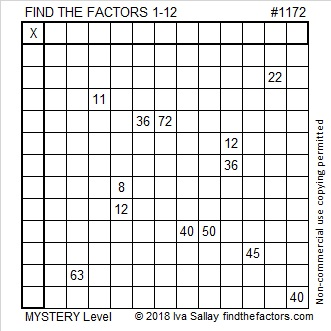# 1172 Mystery Puzzle

There are thirteen clues in this Mystery Level Find the Factors 1 – 12 puzzle. Will those thirteen clues bring you good luck or bad? The logic needed to solve the puzzle may be a bit complicated, but if you stick with it, you will figure it out. Good luck to you!Print the puzzles or type the solution in this excel file: 12 factors 1161-1173

Let me share some facts about the number 1172:

• 1172 is a composite number.
• Prime factorization: 1172 = 2 × 2 × 293, which can be written 1172 = 2² × 293
• The exponents in the prime factorization are 2 and 1. Adding one to each and multiplying we get (2 + 1)(1 + 1) = 3 × 2  = 6. Therefore 1172 has exactly 6 factors.
• Factors of 1172: 1, 2, 4, 293, 586, 1172
• Factor pairs: 1172 = 1 × 1172, 2 × 586, or 4 × 293
• Taking the factor pair with the largest square number factor, we get √1172 = (√4)(√293) = 2√293 ≈ 34.234491172 is the sum of six consecutive prime numbers:
181 + 191 + 193 + 197 + 199 + 211 = 1172

34² + 4² = 1172

1172 is the hypotenuse of a Pythagorean triple:
272-1140-1172 calculated from 2(34)(4), 34² – 4², 34² + 4²
It is also 4 times (68-285-293)

1172 is a palindrome in a couple of bases:
It’s 818 in BASE 12 because 8(12²) + 1(12) + 8(1) = 1172,
and 494 in BASE 16 4(16²) + 9(16) + 4(1) = 1172

This site uses Akismet to reduce spam. Learn how your comment data is processed.